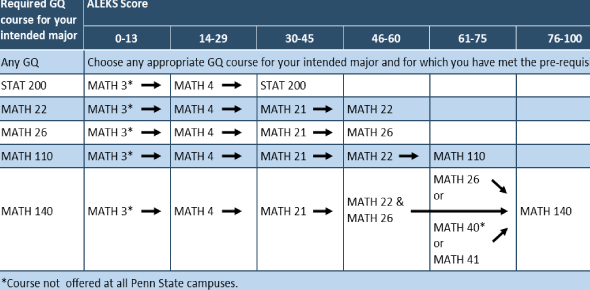# Math Pre-assessment Evaluation

20 Questions | Total Attempts: 39SettingsThis quiz goes over what areas of opportunity need to be worked on in preparation for the extrance exam. TAKE NOTE: Of what you get incorrect so we can see what to work on, it will show you on the top left if you got the last answer correct or incorrect.

Related Topics
• 1.
• 2.
Evaluate(-23) - (-94)
• A.

117

• B.

-117

• C.

-63

• D.

71

• E.

I am not sure how to answer this question

• 3.
Solve & Simplify (Division problem)6(6+5) + 6(3)      =      ____________8(4-1)
• A.

7/2

• B.

84/24

• C.

16

• D.

26/13

• E.

I am not sure how to answer this question

• 4.
Charles drove 630 miles of a 900 miles trip. What percentage of the trip did he drive?
• A.

64%

• B.

70%

• C.

53%

• D.

30%

• E.

I am not sure how to answer this question

• 5.
Reduce to lowest terms.28/42
• A.

2/3

• B.

Can not be reduced further

• C.

14/21

• D.

7/14

• E.

I am not sure how to answer this question

• 6.
What is 6% of 360?
• A.

21.6

• B.

.66

• C.

78.47

• D.

18.6

• E.

I am not sure how to answer this question

• 7.
Multiply -6(-3)
• A.

18

• B.

9

• C.

-18

• D.

-9

• E.

I am not sure how to answer this question

• 8.
Choose the expression that is equivalent to 30x+10y
• A.

5(15x+3y)

• B.

40xy

• C.

5(2x+6y)

• D.

5(6x+2y)

• E.

I am not sure how to answer this question

• 9.
Solve for x and y:2x+y=8x+y=6
• A.

X= 2 y=4

• B.

X= 8 y=6

• C.

X= -2 y=-4

• D.

X= 6 y=8

• E.

I am not sure how to answer this question

• 10.
Solve I4I  -  I-3I =
• A.

-1

• B.

7

• C.

1

• D.

-7

• E.

I am not sure how to answer this question

• 11.
Solve for xx/2   =    12
• A.

6

• B.

14

• C.

24

• D.

-6

• E.

I am not sure how to answer this question

• 12.
Solve for x2/x = 12
• A.

6

• B.

24

• C.

1/6

• D.

-2

• E.

I am not sure how to answer this question.

• 13.
Convert the following into a regular number:4.55 × 10 -1 =
• A.

4.55

• B.

45.5

• C.

455

• D.

.455

• E.

I am not sure how to answer this question

• 14.
Convert this number into scientific notation.35100000 =
• A.

351 x 10(4th power)

• B.

3.51 × 10(7th power)

• C.

.351 x 10(8th power)

• D.

.0351 x10(3rd power)

• E.

I am not sure how to answer this question

• 15.
Find the mean, median, and mode for the number below.7,9,5,3,15,15
• A.

7,14,15

• B.

7,8,15

• C.

8,9,15

• D.

9,8,15

• E.

I am not sure how to answer this question

• 16.
Find the equation of the line that passes through point (8,2) and has a slope of 2
• A.

Y=3x-8

• B.

Y=4x-2

• C.

Y=8x+2

• D.

Y=2x-7

• E.

I am not sure how to answer this question

• 17.
Factor Completelyx² − 9
• A.

(x + 1)(x − 9)

• B.

(x + 3)(x − 3)

• C.

(x - 3)(x − 3)

• D.

(x + 3)(x + 3)

• E.

I am not sure how to answer this question

• 18.
Write the final product(3y + 7)(3y − 7)
• A.

3y² − 7

• B.

9y² + 49

• C.

9y² + 7

• D.

9y² − 49

• E.

I am not sure how to answer this question

• 19.
1 ÷ 1 __ __ 2 6Solve:
• A.

6

• B.

-1/2

• C.

1/6

• D.

3

• E.

I am not sure how to answer this question

• 20.
Simplify2 3 × 2 5
• A.

4(to the power of 8)

• B.

4(to the power of 15)

• C.

2(to the power of 8)

• D.

2(to the power of 15)

• E.

I am not sure how to answer this question